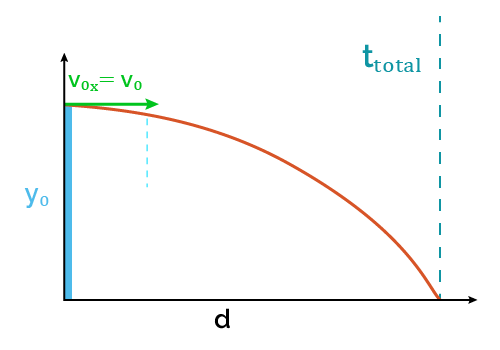# Horizontal Projectile Motion Calculator

Created by Luis Hoyos
Last updated: Aug 06, 2022

Welcome to the horizontal projectile motion calculator, a tool designed to calculate the motion of horizontally thrown projectiles, i.e., with a specific horizontal velocity and zero initial vertical velocity.

With this calculator, you can find any of the following parameters:

In the following sections, you can look at the formulas for horizontal displacement, time of flight, and projectile trajectory.

🙋 Remember, this calculator describes the motion of projectiles whose initial vertical velocity is zero. If you need a calculator that includes the other velocity component, use our projectile motion calculator, where you'll also find the vertical motion formulas.

## Time of flight

The formula to calculate the time of fight is:

ttotal = [V0y + √(V0y² + 2gy0)]/g

where:

• ttotal — Time of flight;
• V0y — Initial vertical velocity;
• g — Gravity acceleration; and
• y0 — Initial height.

As we're dealing with horizontal projectile motion (V0y = 0), the formula reduces to:

ttotal = √(2y₀/g)

From the formula, we can note that, for horizontal projectile motion, the time of flight depends only on the initial height. A higher initial height, as expected, implies more time of flight.

Now, let's see the formula for the horizontal distance traveled by a projectile.

## Horizontal displacement formula

Distance is velocity multiplied by time, and horizontal projectile motion is not the exception. The formula for the horizontal distance traveled by a projectile is:

d = Vxttotal

where:

• d — Horizontal distance traveled; and
• Vx Horizontal velocity.

In projectile motion, we neglect the air resistance, so the horizontal velocity is assumed to be constant (until the object hits the ground), and we can use its value at any given moment.

If you're asking how to calculate horizontal velocity, solve for Vx in the previous equation:

Vx = d/ttotal

Now that we know how to calculate the horizontal velocity, distance traveled, and time of flight, let's look at how to calculate the horizontal projectile motion trajectory.

## Formula to calculate horizontal projectile motion trajectory

When we say trajectory, we refer to the graphical representation of the projectile path, which follows a parabolic trajectory. An example is the water jet in the following image:

The formula that mathematically describes this trajectory is:

$y = y_0 + x\tan{\alpha} - \frac{g x^2}{2 V_\text{0} ^2 \cos^2{\alpha}}$

where:

• $y$ — Vertical position (height) for a corresponding horizontal position;
• $x$ — Horizontal position;
• $y_0$ — Initial height;
• $\alpha$ — Angle of launch; and
• $V_0$ — Initial velocity.

As in horizontal projectile motion $\alpha = 0$, the formula reduces to:

$y = y_0 - \frac{g x^2}{2 V_\text{0} ^2}$

Hopefully, these equations have given you a sounder idea of how this horizontal projectile motion calculator works. Way no more, and use them to double-check the results obtained with the calculator and take your understanding to the next level!

Luis HoyosInitial velocity (V₀)
ft/s
Initial height (y₀)
ft
Time of flight (tₜₒₜₐₗ)
sec
Distance (d)
ft
People also viewed…

### Acceleration due to gravity

Learn how to calculate the acceleration due to gravity on a planet, star, or moon with our tool!

### Magnitude of acceleration

Quickly calculate the magnitude of acceleration in three different ways with CalcTool.